Home > CC3 > Chapter 9 > Lesson 9.1.4 > Problem9-45

9-45.
1.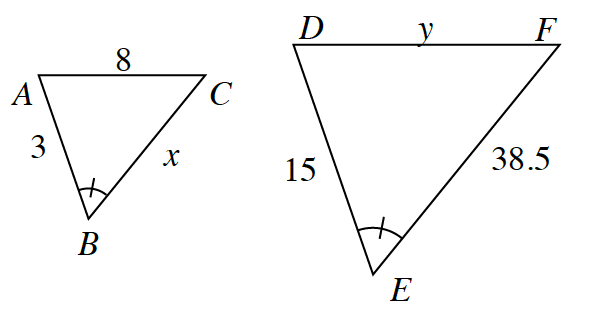ΔABC is similar to ΔDEF. Homework Help ✎

1. Find the scale factor from ΔABC to ΔDEF.

2. Find x.

3. Find y.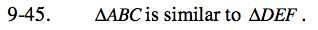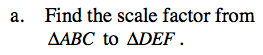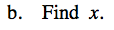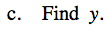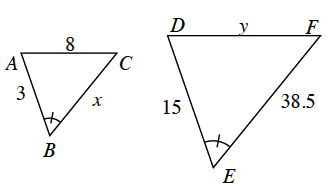Find the scale factor between two given similar sides for the two shapes.

The scale factor is 5.

Divide the side corresponding to side x by the scale factor.

$x=\frac{38.5}{5}$

Multiply the matching side by the scale factor.